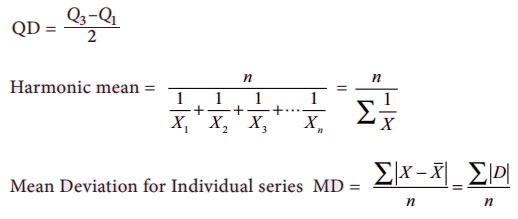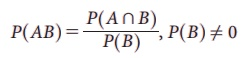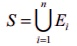Home | | Business Maths 11th std | Summary of Descriptive statistics and probability

# Summary of Descriptive statistics and probability

A measure which divides an array into four equal parts in known as quartiles.

Summary

A measure which divides an array into four equal parts in known as quartiles.

A measure which divides an array into ten equal parts is known as deciles.

A measure which divides an array into hundred equal parts is known as percentiles

Q2 = D5 = P50 = Median

Inter quartile range = Q3 â€“ Q1The conditional probability of an event A given the occurrence of the event B is given byBayeâ€™s Theorem:

If E1 , E2 , E3 ,..., En  are a set of n mutually exclusive and collectively exhaustive events with P(Ei) â‰  0 (i =1,2,3â€¦,n)n, then for any arbitrary event A which is associated with sample spacesuch that P(A) > 0, we haveTags : Probability , 11th Business Mathematics and Statistics(EMS) : Chapter 8 : Descriptive statistics and probability
Study Material, Lecturing Notes, Assignment, Reference, Wiki description explanation, brief detail
11th Business Mathematics and Statistics(EMS) : Chapter 8 : Descriptive statistics and probability : Summary of Descriptive statistics and probability | Probability